Courses

# Test: Dimensional And Model Analysis, Centrifugal Pump - 2

## 30 Questions MCQ Test RRB JE for Mechanical Engineering | Test: Dimensional And Model Analysis, Centrifugal Pump - 2

Description
This mock test of Test: Dimensional And Model Analysis, Centrifugal Pump - 2 for Mechanical Engineering helps you for every Mechanical Engineering entrance exam. This contains 30 Multiple Choice Questions for Mechanical Engineering Test: Dimensional And Model Analysis, Centrifugal Pump - 2 (mcq) to study with solutions a complete question bank. The solved questions answers in this Test: Dimensional And Model Analysis, Centrifugal Pump - 2 quiz give you a good mix of easy questions and tough questions. Mechanical Engineering students definitely take this Test: Dimensional And Model Analysis, Centrifugal Pump - 2 exercise for a better result in the exam. You can find other Test: Dimensional And Model Analysis, Centrifugal Pump - 2 extra questions, long questions & short questions for Mechanical Engineering on EduRev as well by searching above.
QUESTION: 1

Solution:

Ans. (a)

QUESTION: 2

Solution:

Ans. (a)

QUESTION: 3

### The following is the arrangement of rotary pumps in descending orderof specific speed at their best efficiency:

Solution:

Ans. (c)

QUESTION: 4

Which one of the following is correct?
In positive displacement pumps, the slip can sometimes be negativewhen the actual discharge is greater than the theoretical discharge.This happens in

Solution:

Ans. (c)

QUESTION: 5

Assertion (A): The efficiency of a pump is generally less than that of aturbine.
Reason (R): Although the losses in the two types of machines are of the same kind, the losses in pumps are more due to eddy and turbulence.

Solution:

Ans. (a) The order of efficiency in pump is 65% but in turbine efficiency is about to
90%. Assertion A is correct. Pump is against nature that so why efficiency is
low.

QUESTION: 6

When the specific speed of the pump is <2600, the type of pump is

Solution:

The specific speed of the pump is given by NS = NQ 1/2 / H3/4 and when NS > 2600, the type of pump is centrifugal pump.

QUESTION: 7

Centrifugal pumps have which of the following advantages?
1. Low initial cost
2. Compact, occupying less floor space
3. Easy handling of highly viscous fluids
Select the correct answer using the code given below:

Solution:

Ans. (a) Centrifugal pumps has low initial cost and low maintenance cost that so
why it is widely used.

QUESTION: 8

A centrifugal pump driven by a directly coupled 3 kW motor of 1450rpm speed is proposed to be connected to another motor of 2900 -rpmspeed. The power of the motor should be:

Solution:

Ans. (d)

QUESTION: 9

Which one of tile following statements is correct?
When a fluid passes from tile inlet to exit of the rotor in a cerltrlfuga1pump, tangential momentum

Solution:

Ans. (a) Through the rotor we are adding energy to the fluid therefore energy
cannot remains unchanged.

QUESTION: 10

Which one of the following types of impeller vanes are most commonlyused in centrifugal type compressors?

Solution:

Ans. (b) The blades of the compressor or either forward curved or backward curved or radial. Backward curved blades were used in the older compressors, whereas the modern centrifugal compressors use mostly radial blades.

QUESTION: 11

A centrifugal pump is started with its delivery valve kept

Solution:

Ans. (b) A centrifugal pump is started with delivery valve fully closed.

QUESTION: 12

An aeroplane is cruising at a speed of 800 kmph at altitude, where theair temperature is 0° C. The flight Mach number at this speed is nearly

Solution:

Ans. (c)

QUESTION: 13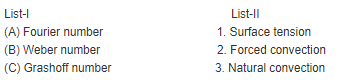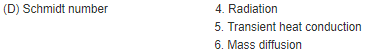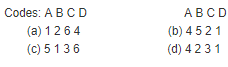Solution:

Ans. (c)

QUESTION: 14

The time period of a simple pendulum depends on its effective length Iand the local acceleration due to gravity g. What is the number of dimensionless parameter involved?

Solution:

Ans. (b) m = 3 (time period, length and acceleration due to gravity); n = 2 (length
and time). Then the number of dimensionless parameter = m – n.

QUESTION: 15

Match List-I (Flow/Wave) with List-II (Dimensionless number) and select the correct answer: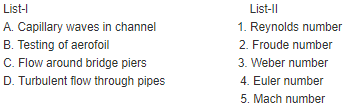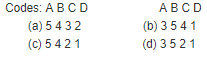Solution:

Ans. (d)

QUESTION: 16

List-I gives 4 dimensionless numbers and List-II gives the types of forces which are one of the constituents describing the numbers. Match List-I with List-II and select the correct answer using the codes given below the lists: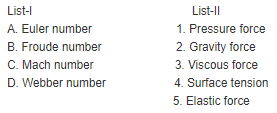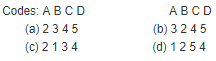Solution:

Ans. (d) Euler number is concerned with pressure force and this choice is available for A in code (d) only. If one is confident, then there is no need to look for items B, C and D. However a cross checks will show that Froude number is concerned with gravity force, Mach number with elastic force, and Weber number with surface tension. Hence the answer is (d) only.

QUESTION: 17

Kinematic similarity between model and prototype is the similarity of

Solution:

Ans. (c) Kinematic similarity between a model and its prototype is said to exist if
the flow patterns are in geometric i.e. velocity, acceleration etc are similar.
Remember discharge is not related with kinematic similarity.

QUESTION: 18

A model test is to be conducted in a water tunnel using a 1: 20 model of a submarine, which is to travel at a speed of 12 km/h deep under seasurface. The water temperature in the tunnel is maintained, so that is kinematic viscosity is half that of sea water. At what speed is the model test to be conducted to produce useful data for the prototype?

Solution:

Ans. (d) Apply Reynolds Model law.

QUESTION: 19

A ship model 1/60 scale with negligible friction is tested in a towing tank at a speed of 0.6 m/s. If a force of 0.5 kg is required to tow themodel, the propulsive force required to tow the prototype ship will be:

Solution:

Ans. (c)

QUESTION: 20

Assertion (A): Reynolds number must be same for the model andprototype immersed in subsonic flows.
Reason (R): Equality of Reynolds number for the model and prototypesatisfies the dynamic similarity criteria.

Solution:

Ans. (b)

QUESTION: 21

A sphere is moving in water with a velocity of 1.6 m/s. Another sphere of twice the diameter is placed in a wind tunnel and tested with airwhich is 750 times less dense and 60 times less viscous than water. The velocity of air that will give dynamically similar conditions is:

Solution:

Ans. (b)

QUESTION: 22

A1:256 scale model of a reservoir is drained in 4 minutes by opening the sluice gate. The time required to empty the prototype will be:

Solution:

Ans. (b)

QUESTION: 23

A 1:20 model of a spillway dissipates 0.25 hp. The corresponding prototype horse power dissipated will be:

Solution:

Ans. (d) Pr = Lr3.5 = 203.5 Therefore Pp = 0.25 × 203.5 = 8944 hp

QUESTION: 24

A model test is to be conducted for an under water structure which each likely to be exposed for an under water structure, which is likelyto be exposed to strong water currents. The significant forces areknown to the dependent on structure geometry, fluid velocity, fluiddensity and viscosity, fluid depth and acceleration due to gravity.Choose from the codes given below, which of the following numbers must match for the model with that of the prototype:
1. Mach number
2. Weber number
3. Froude number
4. Reynolds number.

Solution:

Ans. (d)

QUESTION: 25

The square root of the ratio of inertia force to gravity force is called

Solution:

Ans. (b)

QUESTION: 26

Consider the following statements:
1. Complete similarity between model and prototype envisages geometric and dynamic similarities only.
2. Distorted models are necessary where geometric similarity is notpossible due to practical reasons.
3. In testing of model of a ship, the surface tension forces are generallyneglected.
4. The scale effect takes care of the effect of dissimilarity between model and prototype.
Which of these statements are correct?

Solution:

Ans. (c) 1 is wrong. Complete similarity between model and prototype envisages
geometric, kinematic and dynamic similarities only. 4 is also wrong. The scale effect takes care of the effect of dissimilarity (size difference) between model and prototype.

QUESTION: 27

Why is multi-staging in centrifugal pumps used?

Solution:

Ans. (b) Centrifugal pumps are able to develop low pressure multi-staging in
centrifugal pumps is done for high head.

QUESTION: 28

Which one of the following statements is relevant to the specific speedof a centrifugal pump?

Solution:

Ans. (a)

QUESTION: 29

Consider the following statements regarding the specific speed of acentrifugal pump:
1. Specific speed is defined as the speed of a geometrically similar pump developing unit power under unit head.
2. At the same specific speed, the efficiency is greater with largercapacity.
3. The specific speed increases with the increase in outer blade angle.
4. The specific speed varies directly as the square root of the pump discharge.
Of these statements:

Solution:

Ans. (b) Specific speed is defined as the speed of a geometrically similar turbine
developing unit power under unit head. BUT specific speed of a pump is defined
as the speed of a geometrically similar pump of such a size that under corresponding  conditions it would deliver unit volume flow of liquid against unit
head. That so why statement 1 is wrong.

QUESTION: 30

Assertion (A): With increase in discharge in a single stage centrifugal pump the BHP goes on increasing but beyond a certain discharge the BHP starts decreasing.
Reason (R): Efficiency of the pump starts decreasing beyond a certain discharge.

Solution:

Ans. (d) A is false and R is true.